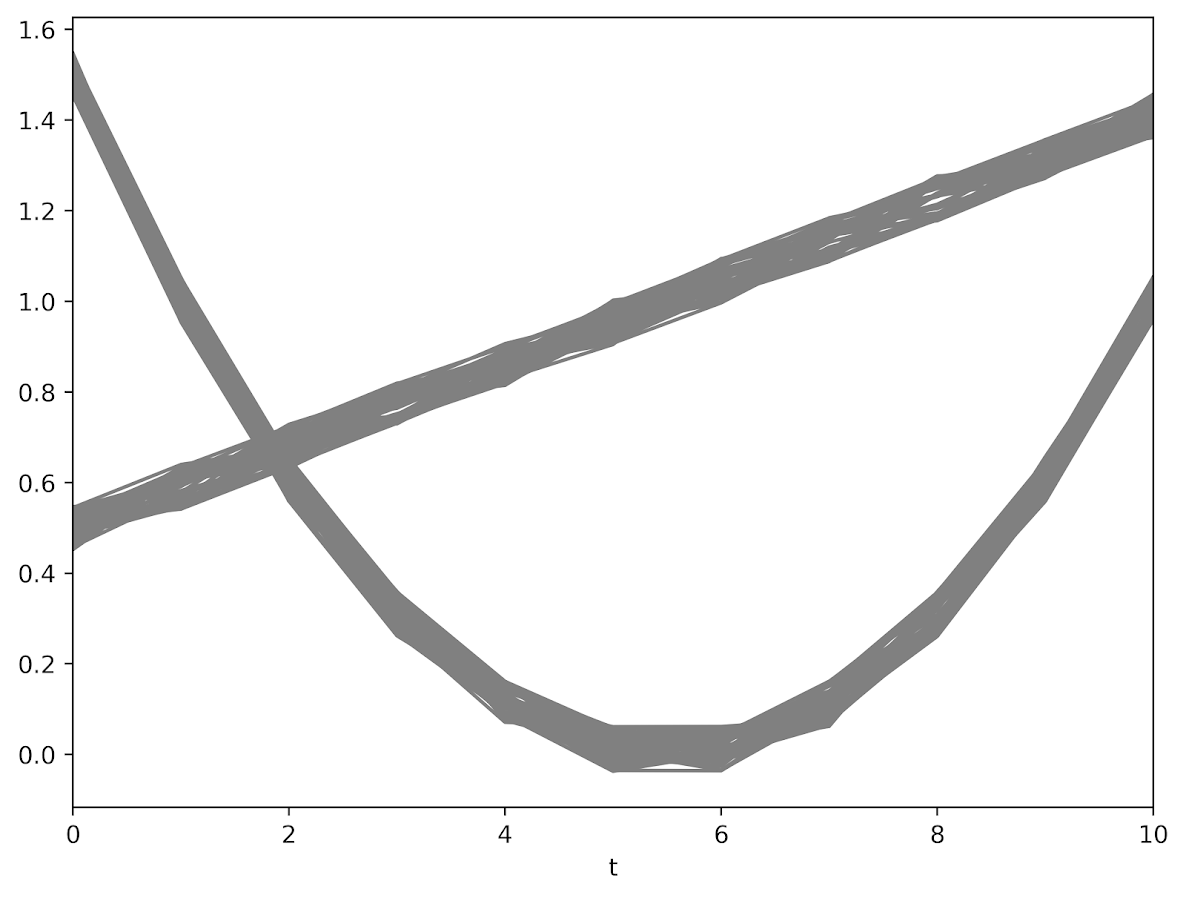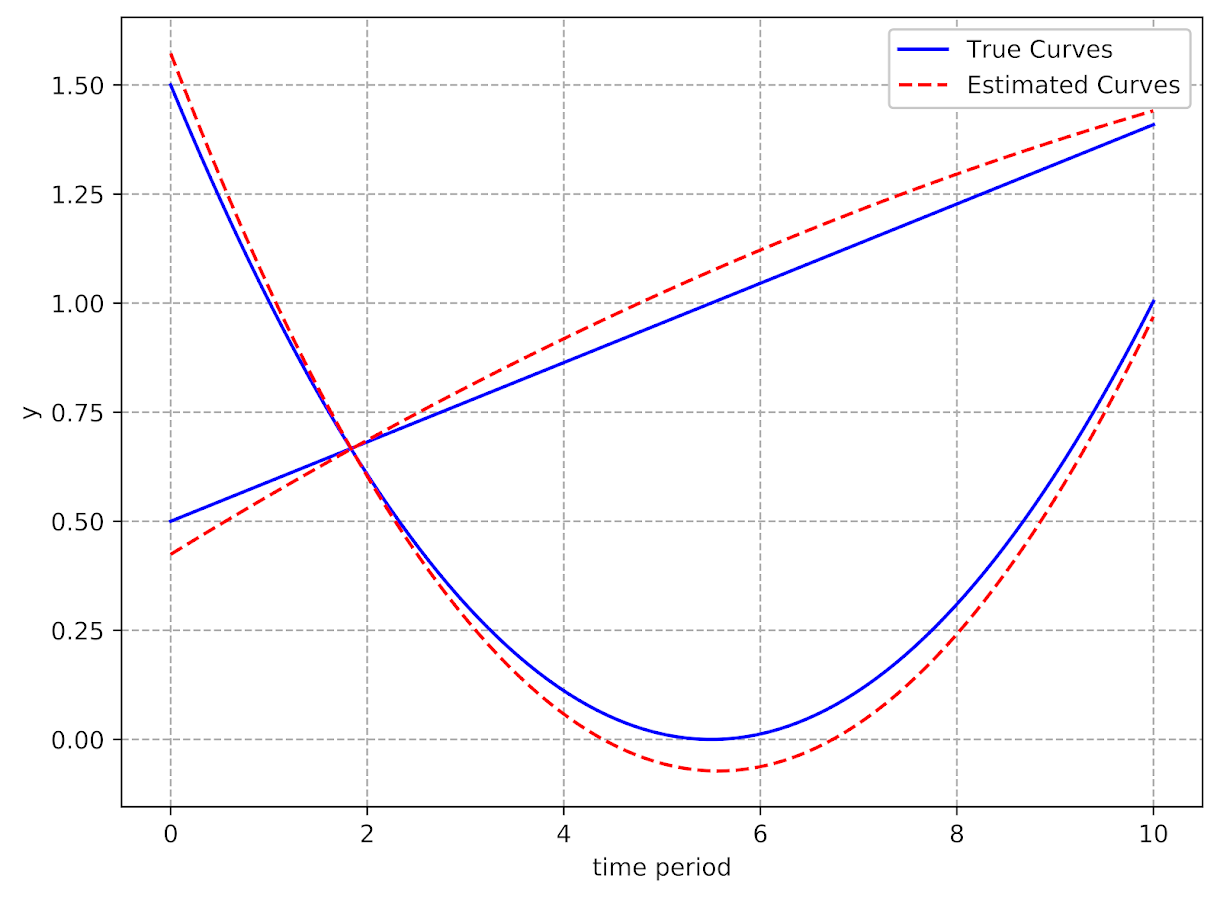# Using pytorch to estimate group based traj models

Deep learning, tensors, pytorch. Now that I have that seo junk out of the way 🙂 – I’ve been trying to teach myself some “Deep Learning”, as it is what all of the cool kids are doing these days.

I was having a hard time though with many of the different examples. Many are for image data, and so it was hard for me to translate that to actual applications I am interested in. Many do talk about dimension reduction and reducing to hidden layers, so I thought that was similar in nature to latent class analysis, such as group-based-trajectory-modelling (GBTM).

If you aren’t familiar with GBTM, imagine a scenario in which you cluster data, and then you estimate a different regression model to predict some outcome for each subset of the clustered data. This is just a way to do that whole set-up in one go, instead of doing each part separately. It has quite a few different names – latent class analysis and mixture modelling are two common ones. The only thing really different about GBTM is that you have repeated observations – so if you follow the same person over time, they should always be assigned to the same cluster/mixture.

In short you totally can do GBTM models in deep learning libraries (as I will show), but actually most examples that I have walked through are more akin to dimension reduction of columns (so like PCA/Canonical Correlation). But the deep learning libraries are flexible enough to do the latent class analysis I want here. As far as I can tell they are basically just a nice way to estimate systems of equations (with a ton of potential parameters, and do it on the GPU if you want).

So I took it as a challenge to estimate GBTM models in a deep learning library – here pytorch. In terms of the different architectures/libraries (e.g. pytorch, tensorflow, Vowpal Wabbit) I just chose pytorch because one of my co-workers suggested pytorch was easier to learn!

I’ve posted a more detailed notebook of the code, but it worked out quite well. So first I simulated two groups of data (50 observations in each group and 11 time periods). I added a tiny bit of random noise, so this (I was hoping) should be a pretty tame problem for the machine to learn.The code to generate a pytorch module and have the machine churn out the gradients is pretty slick (less than 30 lines total of non-comments). Many GBTM code bases make you do the analysis in wide format (so one row is an observation), but here I was able to figure out how to set it up in long data format, which makes it real easy to generalize to unbalanced data.It took quite a few iterations to converge though, (iterations were super fast, but it is a tiny problem, so not sure how timing will generalize) and only converged when using the Adam optimizer (stochastic gradient descent converged to an answer with a similar mean square error, but not to anywhere near the right answer). These models are notorious for converging to sub-optimal locations, so that may just be an intrinsic part of the problem and a good library needs to do better with starting conditions.

I have a few notes about potential updates to the code at the end of my Jupyter notebook. For count or binomial 0/1 data, that should be a pretty easy update. Also need to write code to do out of sample predictions (which I think I can figure out as well). A harder problem I am not sure how to figure out is to do an equation for the latent groups inside of the function. And I don’t know how to get standard errors for the coefficient estimates. Hopefully I can figure that out while trying to teach myself some more deep learning. I have a few convolution ideas I want to try out for spatial-temporal crime forecasting and include proactive police feedback, but I won’t get around to them for quite awhile I imagine.